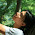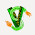## Wednesday, March 07, 2012

### When a+b is harder than b+a

Is 1+4 a harder calculation than 4+1? It may be if you are 2+3 years old. I asked my 7-2 year old great niece Noelle the following sequence of questions. I include how long it took her to answer.

Bill: How old are you?

Noelle: (2 seconds) 5

Bill: What is 3+2

Noelle: (3 seconds) 5

Bill: What is 2+3

Noelle: (2 seconds) 5

Bill: What is 4+1

Noelle: (1 seconds) 5

Bill: What is 1+4

Noelle: (8 seconds) 5

Bill: What is 7 take away 2

Noelle: (4 seconds) 5

Bill: What is the least d such that there is general dth-degree equation.

Noelle: (11 years) 5

Bill: What is the least k such that the kth Ramsey Number is not known

Noelle: (21 years) 5

The most interesting of these to me was that 1+4 took much longer than 4+1. Why is this? To do 1+4 she starts at 1 and adds 1 to it four times so its 1 + 1+ 1+ 1+ 1. To do 4+1 she starts with 4 and adds 1 once, 4+1. More generally, if we don't use the addition is commutative and we view +1 our basic operation than the complexity of a+b is b.

It is important to realize that concepts such as commutativity of addition which are now obvious to us as adults, there was a time when it was not obvious. Or perhaps not obviously useful for calculations.

Could a model of children's addition be defined and studied? Would this be a Math Project, a Math Ed Project, or a Child-Development Project? Has it already been done? I suspect that how children learn things has been studying extensively, but that well defined questions of complexity-of-children's-addition has not. My ONE data point suggests that the complexity of a+b is b, but to really study this you would of course need more samples. SO, if any of you relatives that are 5 or under (but can talk), and try this out, let me know what you find.

1.The phenomenon you described is called the Problem Size effect, and is the subject of several cognitive models. Christian Lebiere's dissertation comes to mind, where the ACT-R cognitive architecture was used to model the time needed to calculate the answer. Like you suggested, iterative counting is one of the back-up strategies, leading to the increased time. This sort of hits the idea of complexity, but from more of a psychology angle than a complexity angle.

2.I seem to remember Stanislaus Dehaene's book, The Number Sense, describes timing tests for multiplication. They can infer up to what number people use table lookup, when they switch over to an algorithmic way to compute product, and which algorithm.

3.This corresponds exactly to my experiments with my 5 (and now 6) year old. And it's precisely for the reason you mention. In fact once he realized how to flip things around, he used one time unit to do the flip, and then did the +1, reducing the complexity to min(a,b)+1 :)

4.1.I also highly recommend Stanislas Dehaene's book "Space, Time and Number in the Brain: Searching for the Foundations of Mathematical Thought".

5.I am concerned that she didn't realize she already had the answer for 1+4 after giving an answer for 4+1 and that she didn't notice the answer is always 5.

6.> The most interesting of these to me was that 1+4 took much
> longer than 4+1. Why is this?

Or maybe she just got confused because you asked for the 5th time something she should answer with a 5. If she smiled or laughed, then I'm pretty sure her kid mind was thinking "what is this silly doing? hihi" :)

7.easy, when b is a limit ordinal and a is a smaller ordinal.

8.Nice experiment! My lay attempt at a theory -
I think our brain evolved to make estimates faster than computing the exact answer. So, when you say "4+1"
(a) 4 enters my brain.
(b) Some of my neurons "know" how large 4 is, in the same sense that logicians train themselves to "know" BB(n), aleph0, aleph1. 4 is a model of something in the world.
(c) 1 enters my brain and _immediately_ my brain makes a comparison i.e knows that the answer isn't very far off from its previous estimate of the real world object, 4.
(d) Addition is performed to verify (b),(c).

When you say "1+4", steps (a),(b) happen the same way, but when (c) happens, instinctive comparison causes my mental estimate of the model to be completely thrown off charts. (d) is performed with no apriori estimate of the answer. New model of reality is created - this causes delay.

When kids learn that addition is commutative, instinctive comparison happens before creating a solid model of the external object and kids start with the bigger number as their initial estimate. "I have 4 sheep and missing 1" is less alarming than "I have 1 sheep and missing 4".

9.The computation time corresponds to a direct aplication of the definition of the addition operation via a+s(b):=s(a+b) and a+0:=a.

Also that 1+4 is not confused with 4+1 (at least for smaller children) corresponds to my experience. And I think this is perfect. A proof that addition is commutative needs the principle of (mathematical) induction. If you remove this principle from the definition of numbers, you have the wanted model. I would say that most humans don't understand the idea of mathematical induction well nor that of a proof, but at some age they anyway start to use commutativity when adding. So they don't do this because of logical understanding, but because they're told that they can use it, or because of the experience they made after doing a lot of calculations (by inductive reasoning :).

10.Is there a natural model of computation, and a natural commutative operation '+', where computing a + b takes different time than computing b + a? (Of course, it would have to be a model where there is non-zero cost for swapping the order of arguments, or perhaps one in which it takes time to decide in the first place whether a + b or b + a is more efficient to compute.)

11.Here’s what I know from Math Ed research:
Very early on, many kids will calculate a + b by counting out a, then counting out b objects, then counting them together, with each counting round starting from 1 (i.e. the number of steps is 2a +2b). The ability to subitize, that is, recognize automatically that, say, 4 chips are four in number without counting out 1, 2, 3, 4 develops eventually but should not be taken for granted. The ability to think about adding numbers without physical objects is also a cognitive leap. In fact, the “mentally counting on from a” strategy used by your daughter represents a significant achievement in addition skill. Other skills include, as you suggested, using the commutative property for efficiency; ideally this is used as a tool in subtraction as well as addition. (For example, calculating 21 – 17 by counting backwards: 20, 19, 18, 17, and stopping there to give an answer of 4, rather than counting back seventeen times 20, 19, 18, …. 4.) At a certain point, kids hopefully develop the skill to count in chunks larger than 1, and use known combinations and an understanding of place value for efficiency. For example, if 2 + 3 is a known fact from memory, then 22+3 can become a single step calculation. Memorized facts can be used together. For example, if I know that 8 + 8 =16, I can determine 8 + 5 by using my known fact followed by jumping back 3 in a single hop. (At this stage, students know that 5 is 3 less than 8 without thinking, and can calculate 16 – 3 without counting backwards by 1’s). Compensation/regrouping should be used strategically also. For example, 28 + 6 = (28 + 2)+ 4 = 34. Interestingly, many adults still don’t do this: If you ask them to add 997 + 505 without a calculator, many will balk or begin doing the standard algorithm with pencil and paper rather than take advantage of the nearness of 997 to 1000.

1.Laura Bofferding12:54 PM, March 08, 2012

This area has been fairly well-documented in the math ed literature as well as some of the child development/psychology literature. Karen Fuson did a lot of work laying out levels of students' counting and addition strategies such as counting all and counting on, which you mentioned. Arthur Baroody did some studies regarding children's use of the commutative property, and Robert Siegler has done some work on modeling students' strategies for solving addition problems. (http://www.psy.cmu.edu/~siegler/shragersiegler98.pdf)

12.John Cherniavsky1:16 PM, March 08, 2012

Bill - children's mathematical reasoning and the inherent number sense of humans (and animals for that matter) have been widely studied (Dehaene was mentioned earlier). The late Robbie Case has a nice developmental theory you might want to look at http://www.psych.ualberta.ca/GCPWS/Case/Biography/Case_bio2.html. These theories are being implemented in teacher education research, for example, in Deborah Ball's research at the University of Michigan http://www-personal.umich.edu/~dball/ . Your research with your niece confirms what's been known for a long time.

13.1+\omega<\omega+1

14.Gasarsch, sometimes i am amazed by why u r so amazed. It's kinda a little retarded if u allow me say to be so amazed.

why is 1+5 more difficult than 5+1 ?

u mean by difficult , why does it take more time for the kiddo to respond to ur question. please correct me if i misunderstood u on this point.

well, for one, it depends, how u count time. assume there to be a correct way that u employed.

then isn't it obvious that a kiddo would naturally be surprised to be hearing, how much is 1+5 ???
and now how much is 5+1 ???
it would enter the loop of testing, wait a minute, this 110 year old (!) grandpa called Billy, is asking me the same question ... but he reversed the order ? why would he wanna do this ? Is this a trick question ? That trick question does not work because these two statements are equivalent. maybe he suffers from alzheimer ? Don't u think it's weird he is posing this ? Does my dad know that grandpa has alzheimer ?

By having naturally undergone this thought process, the kid has spent an additional 3 seconds.

QED.

15.This is a great post!

This completely brings up an interesting concept. The non-commutative nature of algorithms. Even though 1+4 - 4+1 = 0 is commutative, this demonstrates that it is possible that the algorithm to solve the equation may not be commutative or rather Algorithm(1+4) != Algorithm(4+1).

So even though the results are the same, the ordering of the inputs will impact the speed of getting to the results. One way to think about it is to imagine adding a lot of data to small that is open versus adding a little data to a large file that is open. The operation of reallocating memory might be dependent on the change in the size of the file. So adding a lot of new memory might take longer than adding a small amount of memory. This gets to a great point of efficiency in processes.

Absolutely great post to think about!

16.Hal, you could also note that addition and multiplication are not associative on floating points numbers, and it's possible to get accuracy improvements by reordering the operands. http://en.wikipedia.org/wiki/Floating_point#Accuracy_problems

17.This is a great post!
A related discussion appeared over n-category cafe
here http://golem.ph.utexas.edu/category/2006/10/knowledge_of_the_reasoned_fact.html